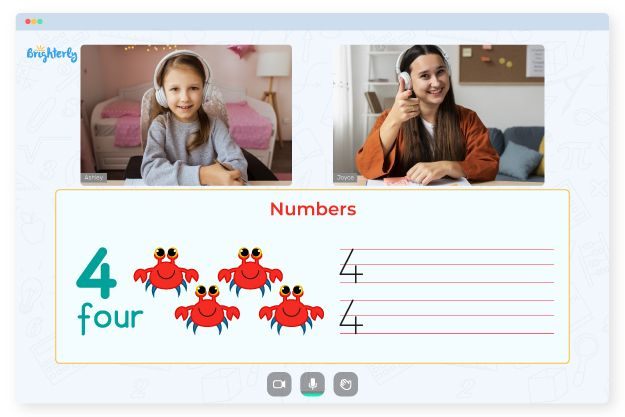# Fact Family Worksheets 1st Grade

Fact families are a fundamental mathematical concept that aids in development of children’s number sense and arithmetic abilities. They are collections of the same-number addition and subtraction facts that are related. As a result, children gain a better understanding of the relationships between numbers and develop the ability to solve problems when they learn about fact families.

First grade fact family worksheets are a great way to help 1st graders master fact families and develop their math skills, and you can use the tools whether you are a teacher or a parent looking for ways to support your child’s math learning at home.

1st grade math facts worksheets are a great way to help students in the first grade understand math fundamentals. A collection of math facts that use the same numbers is called a fact family. The purpose of math facts worksheets 1st grade is to help students understand how multiplication and division relate to addition and subtraction.

Math for Kids

Is Your Child Struggling With Math?
1:1 Online Math Tutoring## Benefits

First grade math facts worksheets are an excellent way to help first-graders understand math fundamentals. Students can build a solid foundation for learning more advanced math concepts in the future by understanding how multiplication and division relate to addition and subtraction. In addition, teachers can use a first grade math facts worksheet to test their student’s understanding of basic math concepts as well as help them practice and become more fluent in those skills.### Fact Family Worksheets 1st Grade PDF### Fact Family Worksheets 1st Grade PDF### Fact Family Worksheets 1st Grade PDF### Fact Family Worksheets 1st Grade PDF

Struggling with Numbers?• Is your child having difficulty with numbers concepts?
• Try lessons with an online tutor.

Does your child struggle with basic numbers? An online tutor could assist.